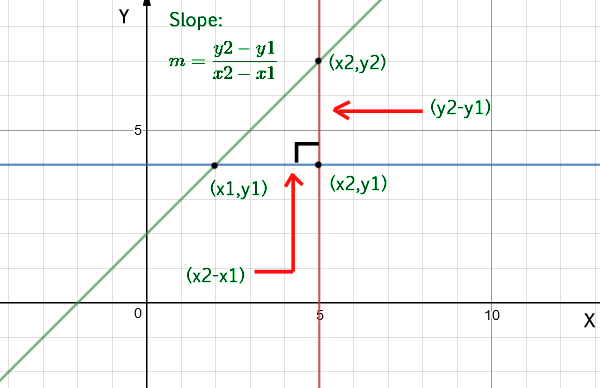# Program to find slope of a line

• Difficulty Level : Easy
• Last Updated : 04 Sep, 2021

Given two coordinates, find the slope of a straight line.

Examples:

Attention reader! All those who say programming isn't for kids, just haven't met the right mentors yet. Join the  Demo Class for First Step to Coding Coursespecifically designed for students of class 8 to 12.

The students will get to learn more about the world of programming in these free classes which will definitely help them in making a wise career choice in the future.

```Input  : x1 = 4, y1 = 2,
x2 = 2, y2 = 5
Output : Slope is -1.5```

Approach: To calculate the slope of a line you need only two points from that line, (x1, y1) and (x2, y2). The equation used to calculate the slope from two points is:Below is the implementation of the above approach:

## C++

 `// C++ program for slope of line``#include ``using` `namespace` `std;` `// function to find the slope of a straight line``float` `slope(``float` `x1, ``float` `y1, ``float` `x2, ``float` `y2)``{``    ``return` `(y2 - y1) / (x2 - x1);``}` `// driver code to check the above function``int` `main()``{``    ``float` `x1 = 4, y1 = 2;``    ``float` `x2 = 2, y2 = 5;``    ``cout << ``"Slope is: "``         ``<< slope(x1, y1, x2, y2);``    ``return` `0;``}`

## Java

 `// Java program for slope of line``import` `java.io.*;` `class` `GFG {``    ``static` `float` `slope(``float` `x1, ``float` `y1,``                       ``float` `x2, ``float` `y2)``    ``{``        ``return` `(y2 - y1) / (x2 - x1);``    ``}``    ``public` `static` `void` `main(String[] args)``    ``{``        ``float` `x1 = ``4``, y1 = ``2``;``        ``float` `x2 = ``2``, y2 = ``5``;``        ``System.out.println(``"Slope is "` `+``                    ``slope(x1, y1, x2, y2));``    ``}``}`

## Python

 `# Python program for slope of line``def` `slope(x1, y1, x2, y2):``    ``return` `(``float``)(y2``-``y1)``/``(x2``-``x1)` `# driver code   ``x1 ``=` `4``y1 ``=` `2``x2 ``=` `2``y2 ``=` `5``print` `"Slope is :"``, slope(x1, y1, x2, y2)`

## C#

 `// C# program for slope of line``using` `System;` `class` `GFG``{``    ``static` `float` `slope(``float` `x1, ``float` `y1,``                       ``float` `x2, ``float` `y2)``    ``{``        ``return` `(y2 - y1) / (x2 - x1);``    ``}``    ` `    ``// Driver code``    ``public` `static` `void` `Main()``    ``{``        ``float` `x1 = 4, y1 = 2;``        ``float` `x2 = 2, y2 = 5;``        ``Console.WriteLine(``"Slope is "` `+``                    ``slope(x1, y1, x2, y2));``    ``}``}` `// This code is contributed by vt_m.`

## PHP

 ``

## Javascript

 ``
Output
`Slope is: -1.5`

Time Complexity: O(1)

Auxiliary Space: O(1)

Special Case: The above code will throw a runtime error when int the function x1 is equal to x2 (x1==x2). In that case, our denominator will become zero(0). To avoid that condition, we will add a condition in the slope function.

## C++

 `// C++ program for slope of line``#include ``using` `namespace` `std;` `// function to find the slope of a straight line``float` `slope(``float` `x1, ``float` `y1, ``float` `x2, ``float` `y2)``{``    ``if``(x1 == x2)``        ``return` `INT_MAX;``    ``return` `(y2 - y1) / (x2 - x1);``}` `// driver code to check the above function``int` `main()``{``    ``float` `x1 = 4, y1 = 2;``    ``float` `x2 = 2, y2 = 5;``    ``cout << ``"Slope is: "``         ``<< slope(x1, y1, x2, y2);``    ``return` `0;``}`

## Java

 `// Java program for slope of line` `import` `java.util.*;` `class` `GFG {` `    ``// function to find the slope of a straight line``    ``static` `float` `slope(``float` `x1, ``float` `y1, ``float` `x2, ``float` `y2) {``        ``if` `(x1 == x2)``            ``return` `Integer.MAX_VALUE;``        ``return` `(y2 - y1) / (x2 - x1);``    ``}` `    ``// driver code to check the above function``    ``public` `static` `void` `main(String[] args) {``        ``float` `x1 = ``4``, y1 = ``2``;``        ``float` `x2 = ``2``, y2 = ``5``;``        ``System.out.print(``"Slope is: "` `+ slope(x1, y1, x2, y2));``    ``}``}` `// This code contributed by Rajput-Ji`

## Python3

 `# Python3 program to find slope``import` `sys` `# Function to find the slope of a straight line``def` `slope(x1, y1, x2, y2):``    ` `    ``if` `x1 ``=``=` `x2:``        ``return` `(sys.maxsize)``        ` `    ``return` `((y2 ``-` `y1) ``/` `(x2 ``-` `x1))` `# Driver code``x1 ``=` `4``y1 ``=` `2``x2 ``=` `2``y2 ``=` `5` `print``(``"Slope is :"``, slope(``4``, ``2``, ``2``, ``5``))` `# This code is contributed by vaishaligoyal878`

## C#

 `// C# program for slope of line``using` `System;``class` `GFG {` `    ``// function to find the slope of a straight line``    ``static` `float` `slope(``float` `x1, ``float` `y1, ``float` `x2, ``float` `y2) {``        ``if` `(x1 == x2)``            ``return` `1000000000;``        ``return` `(y2 - y1) / (x2 - x1);``    ``}` `    ``// driver code to check the above function``    ``public` `static` `void` `Main(``string``[] args) {``        ``float` `x1 = 4, y1 = 2;``        ``float` `x2 = 2, y2 = 5;``        ``Console.Write(``"Slope is: "` `+ slope(x1, y1, x2, y2));``    ``}``}` `// This code is contributed by famously.`

## Javascript

 ``
Output
`Slope is: -1.5`

Time Complexity: O(1)

Auxiliary Space: O(1)

My Personal Notes arrow_drop_up